# Partial Fulfilment of the Requirements for the Degree of

N/A
N/A
Protected

Share "Partial Fulfilment of the Requirements for the Degree of "

Copied!
240
0
0

Full text

Thus, a parameter-based approach is followed for the study and analysis of this latter system. In the current thesis, an attempt was also made to perform both linear and non-linear stability analyzes of the channel.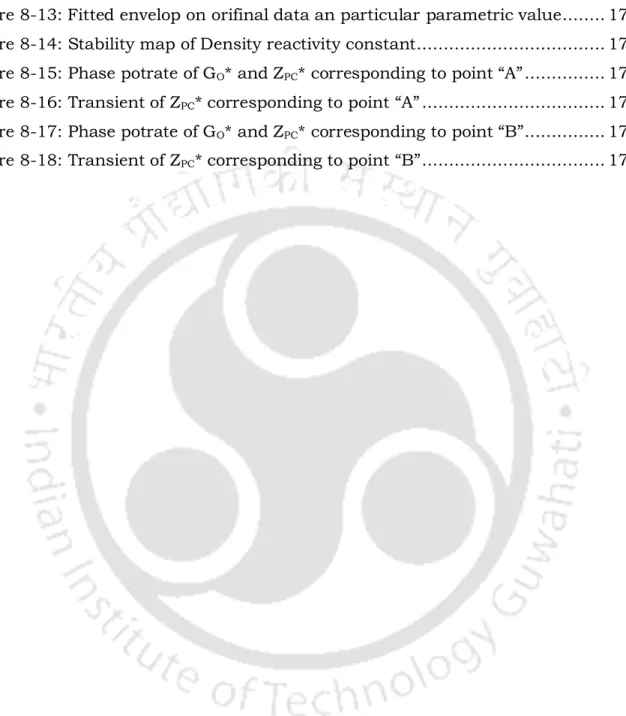Figure 8-12: Transient Ground acceleration plot using Kania-Tajimi power spectral density ...........................................................................................................

## Motivation

This provides a reduction in the size of the reactor coolant pumps, pipes and related equipment, and a reduction in the pumping power. A lower coolant mass supply results from the one-time coolant path in the reactor vessel.

## Classification of flow instability

### Static instability

Pressure drop characteristics of a flow channel, nucleation characteristics and flow regime transitions play an important role in characterizing static instabilities. Where P is the steady pressure drop along the flow channel, and G is the mass velocity.

### Dynamic instabilities

In addition, it is characterized by a sudden change in flow to a lower value or a reversal of flow. Roughly speaking, disturbances are carried by two types of waves: pressure or acoustic waves and void or density waves.

Coupled neutronics instabilities

Mathematical modelling

## Theoretical analysis of instability

### Real time analysis

Furthermore, a large number of calculations should be run to perform the parametric study of the system. However, with increasing computing potential, it is definitely a promising method as the nonlinearity of the system is also taken into account in this approach.

### Linear stability analysis

The system is said to be unstable if this perturbation grows to a sustained or divergent oscillation with the evolution of time. Time domain analysis is generally computationally very expensive due to the strict small step constraints.

### Nonlinear stability analysis

TPFM-Two phase fluid model, DFM-Drift flux model, HEM-Homogeneous equilibrium model, DFM-Drift flux model, P-K-Point kinetics, M-P-K-Multiple point kinetics.

Instability due to seismic effect

Research objectives

## Outline of the thesis

After completed two-zone stability analysis, three-zone stability analysis should be done to predict the effect of increasing the number of zones. The main focus of this chapter is to perform stability analysis using large perturbation (non-linear stability analysis).

## Instability in SCWR

The paper presents the information of the test loop and the numerical analysis model of the loop. Based on the results of the first series of measurements, it was established that the measured part of the stationary state characteristic is independent of the system pressure.

## Instability in BWR

The work performed here describes the application of TRACE/PARCS to all stability test points from cycle 14 of the Ringhals benchmark. Then, a linear stability analysis is performed in the frequency domain to establish the stability map of a natural circulation loop. 2013) is about modeling local instabilities in the Boiling Water Reactor (BWR) via so-called Reduced Order Models (ROMs).

## Scaling for instability of fluid in SCWR

They then applied the four sets of scaling laws to this data to obtain values ​​of the water-equivalent heat transfer coefficient and. Changes in the integrated effect of the specific heat have been used to explain changes in the heat transfer coefficient due to changes in the applied heat flux.

## Mathematical modelling

The program system can be used to optimize the axial enrichment profile of the fuel rods. The calculation results indicated that the decay ratio of the first current path varies monotonically with the power and flow.

## Seismic effect on flow instability

The behavior of the core and the coolant was calculated in the different acceleration parameters. The moving time window technique was used to evaluate the time-varying parameters of the model using actual earthquake records.

## Observation from literature survey

Despite the above facts, there is a need for a simplified simulator/program that quickly captured the above phenomena. A scaled-down version of the HPLWR using Freon R23 as scaling fluid, DeLight, was built at the Delft University of Technology.

## Introduction

The mass and energy equations are integrated along the first node, i.e. from the inlet to the appearance of the pseudocritical boundary. By reintegrating the conservation equations over the second node, from the pseudocritical boundary to the channel exit, we obtain two ODEs with respect to the exit enthalpy.

## Lumped parameter model

### Thermal-hydraulic modelling

The choice of the reference pressure (𝑝𝑟) is quite arbitrary, and therefore the channel outlet pressure is chosen as a reference here, so that the pressure drop across the channel (∆𝑝) can be equated with the input value only in the non-dimensional scale. They can be seen to be analogous to the subcooling number and the phase change number for a boiling channel, respectively.

### Fuel dynamics

The average coolant temperature for each node is taken as the mean of the inlet and outlet values ​​for that node. Using the nondimensional definitions proposed in Eq. 3.4), dimensionless forms of the two equations above can be given as:

### Power dynamics

Therefore, the energy balance of the fuel rod, characterized by a constant steady-state power (Pss), can be presented as,. By setting the steady-state reactivity to zero and neglecting the time dependence, the feedback reactivity can be correlated to the fuel rod temperature and coolant enthalpy as, .

### Comparison of steady state results

In their model, different pressure drop coefficients of the inlet and outlet port were used to match the stable axial pressure distribution they obtained. The comparison of the steady-state result at a power input of 25 kW/m obtained by LPM with that of the model of Ambrosini and Sharabi (2008) is shown in Figure 3-2 and Figure 3-3.

## Transient equations

### Pressure drop boundary condition

The above two ODEs can be combined to obtain the following algebraic equation of the input mass flux. Similar to the first node, the above two ODEs can also be combined into a single equation as, .

### Mass flow rate boundary condition

Therefore, the initial system of PDEs is converted into a series of algebraic equations and ODEs, with dimensionless time (𝑡∗) as the only independent variable. That eliminates the complexity associated with solving a system of PDEs and eliminates the enormous computational power required for finite difference simulation.

## RELAP model

### Nodal sensitivity test

The influence of nodalization on the system stability has been investigated by performing a sensitivity analysis on the RELAP model. Based on these observations, a stability map, Figure 3-6, has been drawn which shows the good agreement between models.

### Comparison of LPM and RELAP5

The disparity with RELAP5 can be attributed to the lumped nature of fluid properties in both areas. The stability of the system is deduced from the nature of the time evolutions of various variables such as the geometric parameter, the operating parameter and the mass flow rate.

## Validation of LPM with the experimental result

According to Xi et al., (2014a) the codes, which were used for comparison with the experiment, could not predict the oscillation period well, there was a significant difference between the numerical simulation and the experiment. This section mainly added to the comparison of numerical results with experiment (Xi et al., 2014b).

## Stability analysis

### Linear stability analysis

This involves linearizing the nonlinear system about the steady state or equilibrium solution of interest and studying the characteristic roots of the linearized system. If one of the eigenvalues ​​has a positive real part, the system is unstable at that time.

### Non-linear stability analysis

Therefore, a nonlinear analysis of the problem is required to determine the nature of the Hopf bifurcation. Furthermore, this analysis helps us obtain the amplitude of the limit cycles originating from the Hopf point.

Introduction

## Fluid-to-fluid scaling methodology

### Conservation equations

The focus of the current study is on scaling the SCWR core to predict its stability behavior. A single heated channel with a constant flow area is selected, with upward flow and uniform heat flux distribution (Figure 3-1). It is interesting to note that the absolute pressure can be freely chosen, as it is not part of the balance equations, and therefore the system pressure is not expected to play any role in the scaling.

Scaling principles

## Selection of fluids

• Scaling rules
• Pressure
• Length of the channel
• Mass flux
• Hydraulic diameter
• Power and inlet temperature

The chosen geometry is vertical, the length of the channel is related to the gravitational forces acting on the system, and therefore the same has been scaled by equating the Froude number between model and prototype. Fuel rod dynamics is considered in the present model to study its effect on system stability.

## Results and discussion

• Comparison with existing scaling method
• Comparison of different model fluids
• Generalized stability map
• Transient analysis of R-134a

The basic comparisons of the scaling criteria here are made by Rhode et al. The scaling rule for enthalpy and density, which is a function of enthalpy, can be derived from the dimensionless conservation equation of state by Rohde et al.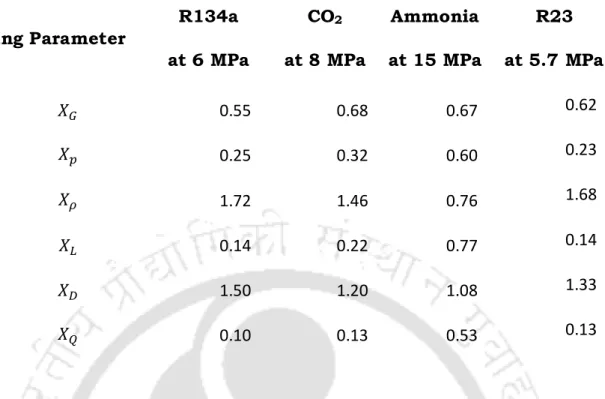Table 4-2: Scaling parameters for different model fluids with water at 25 MPa

## Conclusions

Accordingly, the SCWR can exhibit a large variation in density across the core, making it sensitive to density wave instability, similar to BWRs (Blázquez et al. 2013; Gajev et al. 2013; Peng et al., 1986), thus emphasizing on passive safety design. However, there are a limited number of studies on the stability response of forced supercritical flow channels, especially with two-phase property variations over a short distance around the pseudocritical point (Marcel et al.

Mathematical modelling

## Results and discussion

### Transients simulations

Time evolution of 𝑧𝑝𝑐∗ for the system near the marginal stable boundary, which is shown by point 'b' in Figure 5-5. At some point 'c' far from the limit cycle, it is detected that with a small increase in the input power, oscillations with increasing amplitude can be observed (Figure 5-6).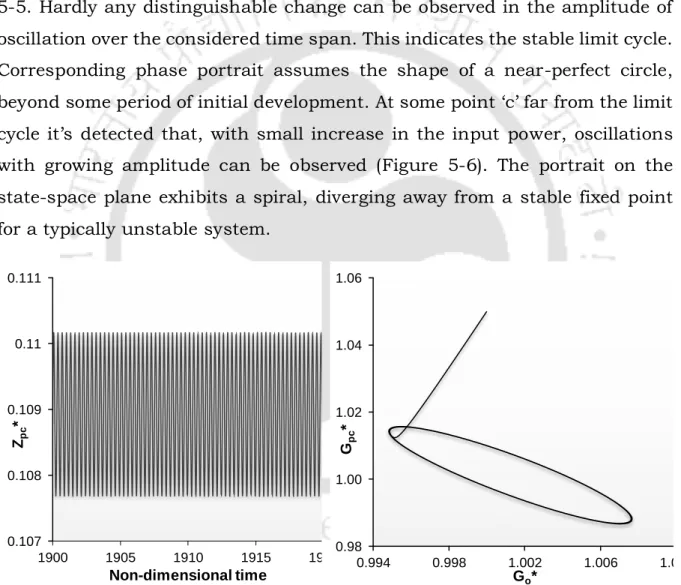Figure 5-5: Neutrally stable response of SCWR corresponding to point ‘b’ in the stability map at 25 MPa system pressure, 1843 kg/s mass flow rate and 0.35 MW/Channel input power

### Parametric effects on pure thermal hydraulic stability

The effect of channel length on the stability threshold is shown in Figure 5-7 and Figure 5-8. Furthermore, the effect of the hydraulic diameter (𝐷ℎ) of the coolant channel on the margins for instability is analyzed.

### Coupled neutronics-thermal hydraulic stability

The fuel time constant, in the present analysis, was varied over a wide range from its normal operating value to study its influence on the stability. Previous studies on BWR (March-Leuba and Rey, 1993; Nayak et al., 2000) have shown that changes in fuel time constant have both stabilizing and destabilizing effects.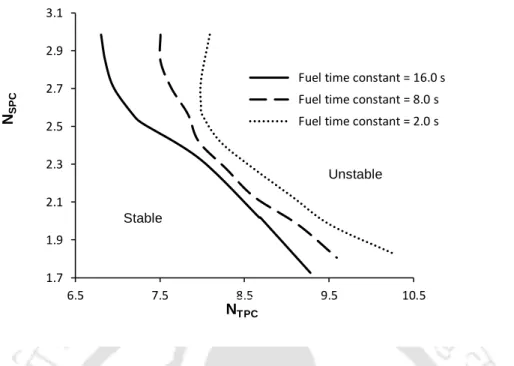Figure 5-13: Effect of Fuel time constant at pressure 25MPa and enthalpy reactivity coefficient is –0.005

## Conclusions

The results reported here predict the stability behavior of the SCWR considering thermal hydraulics, fuel rod dynamics, and force dynamics.

## Introduction

Regarding the previous studies, as mentioned above, the three commonly used mathematical models for the study of density wave instabilities of SCW flows are carried out (Zhang et al., 2015; Zuber, 1966), which are based on the frequency domain method. Many efforts have been made in recent decades (Zhang et al., 2015; Zuber, 1966) and several models have been established for the investigation of the density wave instability of SCW in pipes based on the models used for subcritical conditions.

## Mathematical modelling

### Equations of state (EOS)

Second, the temperature at the exit boundary between H-L-M and L-F is somewhat low, which is approximately 450 oC. These equations of state for each of the regions are obtained by fitting a piecewise linear function with IAPWS data for water at 25MPa (Figure 6-2) given as below.

### Enthalpy profile and relation between 𝝆 ∗ and 𝒉 ∗

Use of property relations in non-dimensional governing equations and integration of the equation for three different areas for the equation of state variables. The mass and energy equations are further integrated in region 1 from the input to Z1, which yields an ODE and an algebraic equation.

## Results and discussion

### Effect of length

For a given inlet temperature, the neutral stability curve shifts towards the lower power level as the channel length increases. This phenomenon can be explained on the basis of gravitational pressure drop and friction in the momentum equation.

### Effect of hydraulic diameter

As the length of the channel increases, the mass flow rate decreases, as the pressure drop through the channel is a predetermined boundary condition. Some irregular shapes in the stability map were observed during the simulations, which are due to the variation of the aperture coefficients as observed in the two-zone model.

### Effects of orifice coefficients

The corresponding neutral stability limit with a hydraulic diameter for a fuel composition of SCWR is given in solid curve. It should be noted that a typical fuel composition of a supercritical American reference design of SCWR has a hydraulic diameter of Dh = 3.4 mm.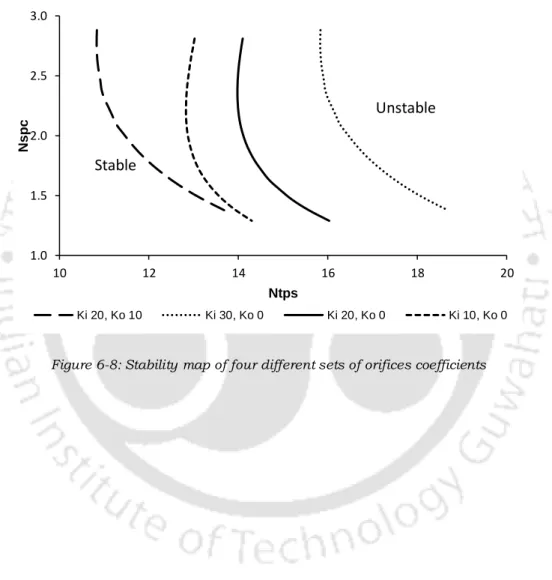Figure 6-8: Stability map of four different sets of orifices coefficients 1.0

Comparisons of two-zone and three-zone model

## Conclusions

Parametric effects on the stability threshold have been investigated and the main conclusions are the following. a) Increasing the heated channel length has a destabilizing effect on the system, which is also quite common in boiling channels. The results reported here predict the stability behavior of the SCWR, considering only the three-zone LPM.

Introduction

Mathematical modelling

## Results and discussion

### Identification of generalized Hopf (GH) points

Therefore, it is predicted that the system will exhibit supercritical (soft and safe) and subcritical (hard and dangerous) Hopf bifurcations, below and above the GH point, respectively.

Numerical simulation analysis in supercritical region

Numerical simulation analysis in subcritical region

## Analysis of neutronics and geometrical parameters

• Effect of fuel time constant
• Effect of the density reactivity constant
• Effect of the length area ratio
• Effect of the mass flow rate

The coolant density coefficient of the SCWR is very small, only ~5% of that for a typical BWR. The reduced sensitivity of the SCWR is due to the fact that most of the moderating power is provided by the water bars, which have small or no density fluctuations (Zhao et al. 2006a).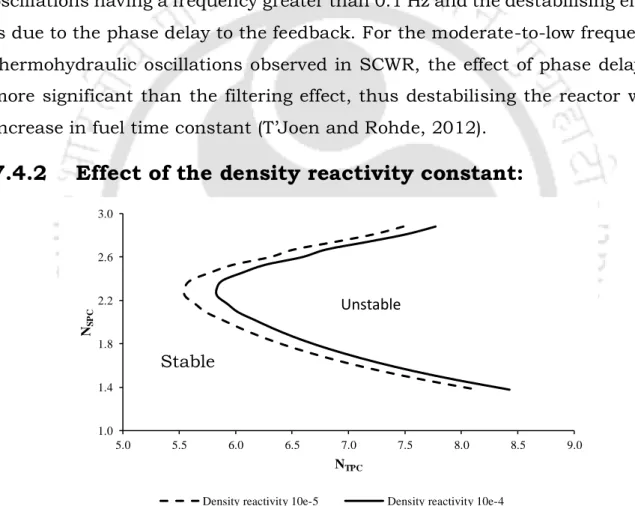Figure 7-8: Stability map of density reactivity constant at 25 MPa and fuel time constant 2s

## Conclusions

Parametric effects on the stability threshold have been investigated and the main results are the following. Increasing the heated channel length and area ratio has a destabilizing effect on the system, which is also quite common in boiling channels.

## Introduction

When a nuclear power plant is subjected to a massive earthquake, the seismic vibration propagates through the internal components of the core, shaking the cooling water with a measurable oscillatory acceleration compared to gravity. They showed that the instability was caused by the resonance between the oscillatory acceleration and the core-wide oscillation, the period of which was about 5 seconds.

Technique & methods

Input model

## Modelling of seismic acceleration

It is difficult to define a realistic interval for the seismic amplitude a∗, since the power spectrum of the amplitude decreases rapidly when the period increases to several seconds, and there are not enough reliable data in this period interval. However, Ishida et al., (1989) formulated a velocity response spectrum assuming Magnitude 8 and an epicenter distance of 50 km for an input seismic motion to study a seismically isolated fixed breeder reactor where the maximum velocity was defined to be 100 kine (cm -s - 1) in the period interval 2-10 s as a conservative value.

## Results and discussion

Sensitivity calculations on the degree of stability and amplitude of

### Neutronics behaviour

With an increase in the density reactivity coefficient, periodic and chaotic oscillations may occur on further evaluations of the effects of different density reactivity feedback coefficients on system stability (Figure 8-11). The results indicate that unstable regions rapidly expand as the density reactivity feedback coefficient increases.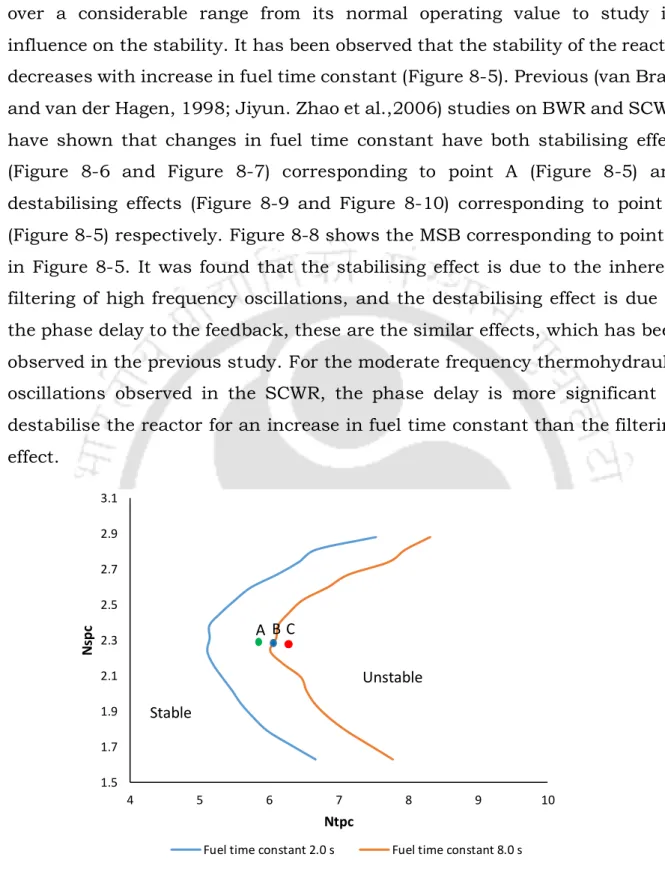Figure 8-5: Stability map of two different Fuel Time Constants 1.5

Kanai–Tajimi model

## Simulation of ground acceleration record

Effect of density reactivity constant

Conclusions

Summary

Conclusions

Future works

Figure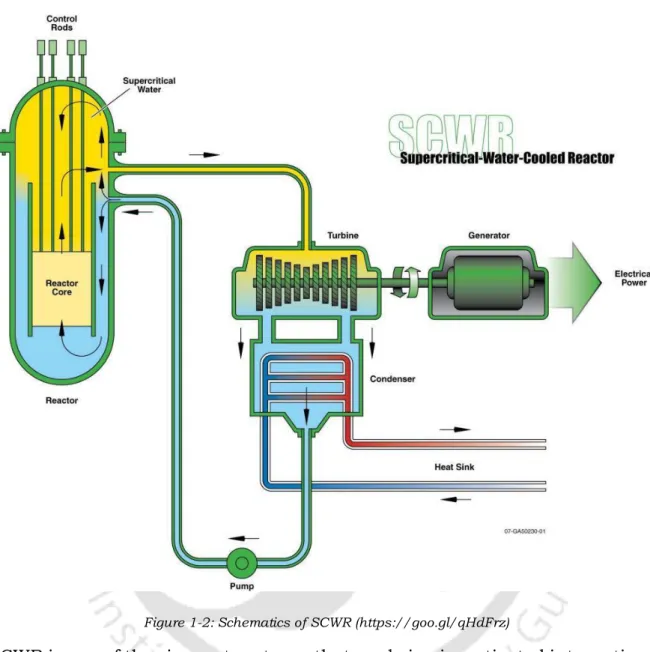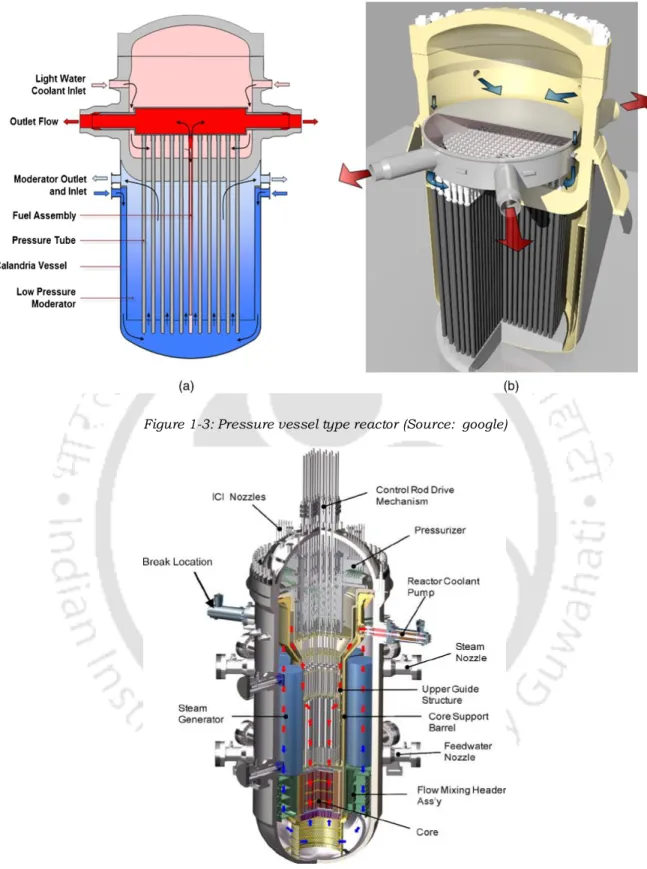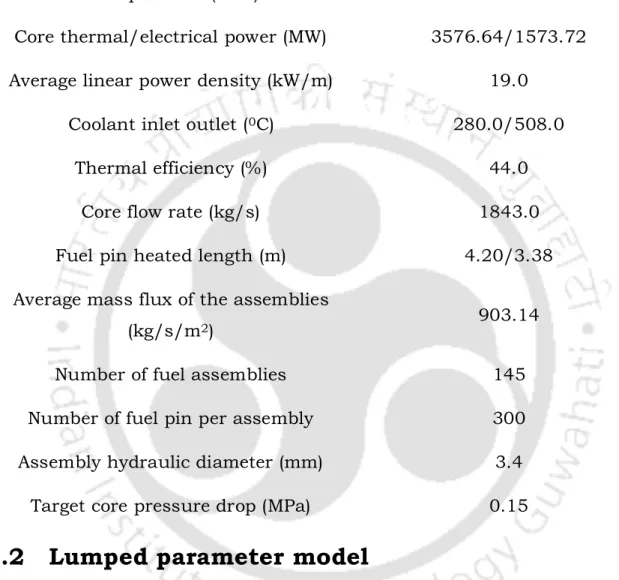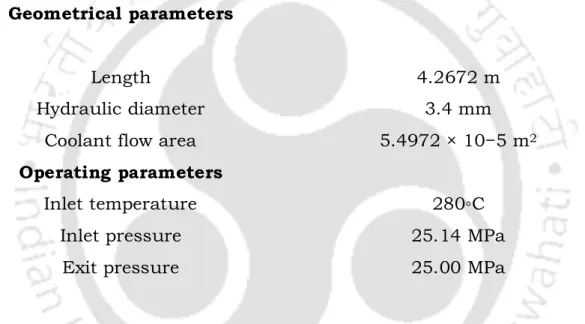+7

References

Related documents

In case the channel is highly correlated for low values offdT , then it is likely that either the first or last frame of the packet will be in error and thus the receiver will not be

The MCGL method is able to model the decision making process of individuals under the framework of MCDA, by revising some of the MCDM mainstream postulates and practices in order to

2.1.2 Chemical Properties The properties of fly ash are strongly influenced by various parameters such as the nature of the coal and the handling, storage and processing methods of

RESULT FOR AL2O3 NANOFLUID The variation in viscosity of nanofluids with Al2O3 as the nanoparticle at different temperatures and volume fractions is shown in Figure 4 to Figure 7... 4

Effect of oscillatory period expressed as n on a the maximum and minimum deformation parameters of inner and outer droplets and the time scale ratio τr, and b the rotation angles

When a laser interacts with a plasma, the emitted photon energy is de- termined by the laser’s intensity, as well as target parameters such as thickness, atomic number, and the cross

The Director, DUFR is in constant contact with the international institutions /organizations such as the Embassies/Consulates of India in foreign countries and helps in updating the

In addition, various parameters such as layer structure, microstructure, magnetic domain structure in multilayer thin films, thickness of spacer and ferromagnetic layers, number of

Cultivars Since cashew is highly cross-pollinated crop, planting of seedlings raised through seed is not recommended.. The improved cultivars are Vengurla-1, Vengurla-4, Anakkayam-l